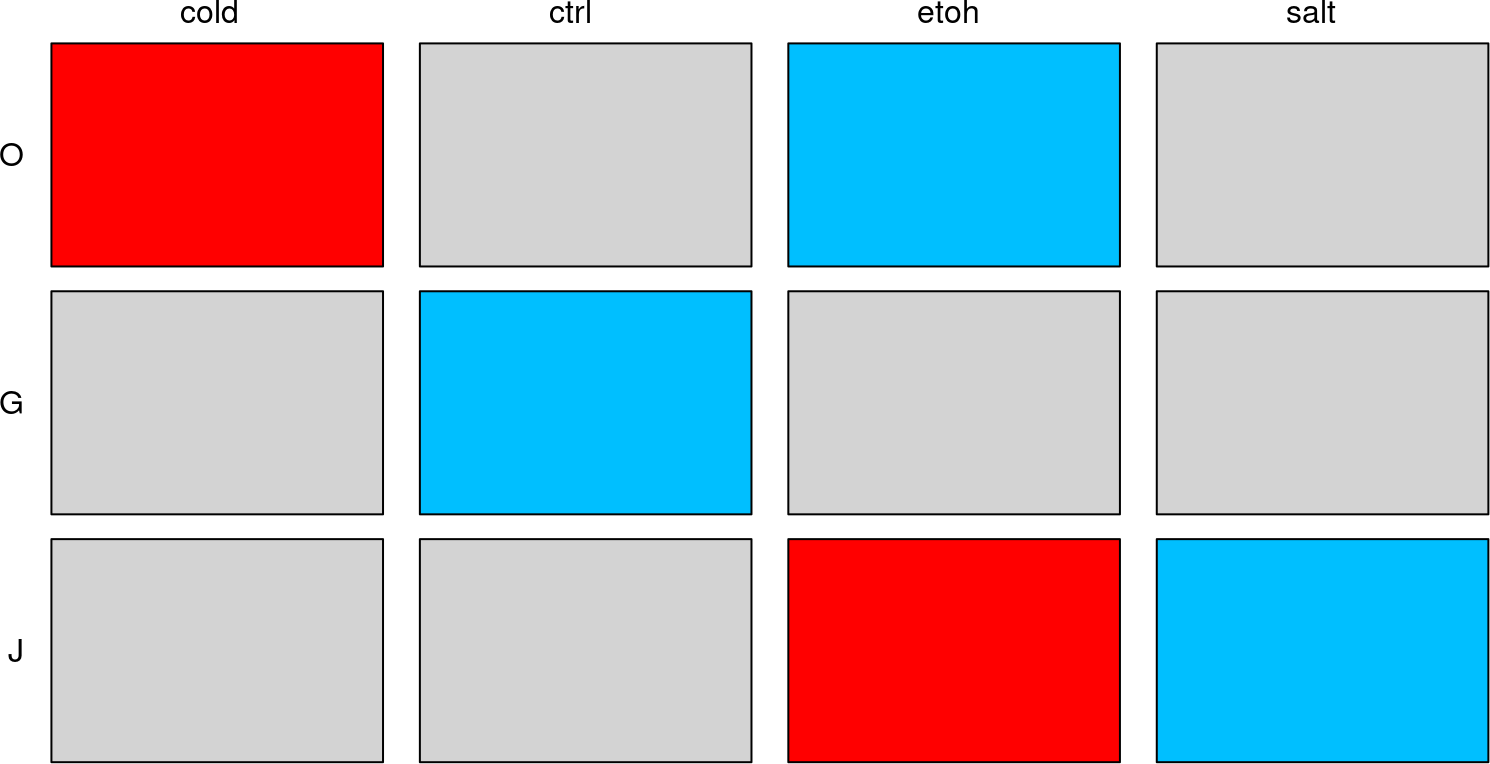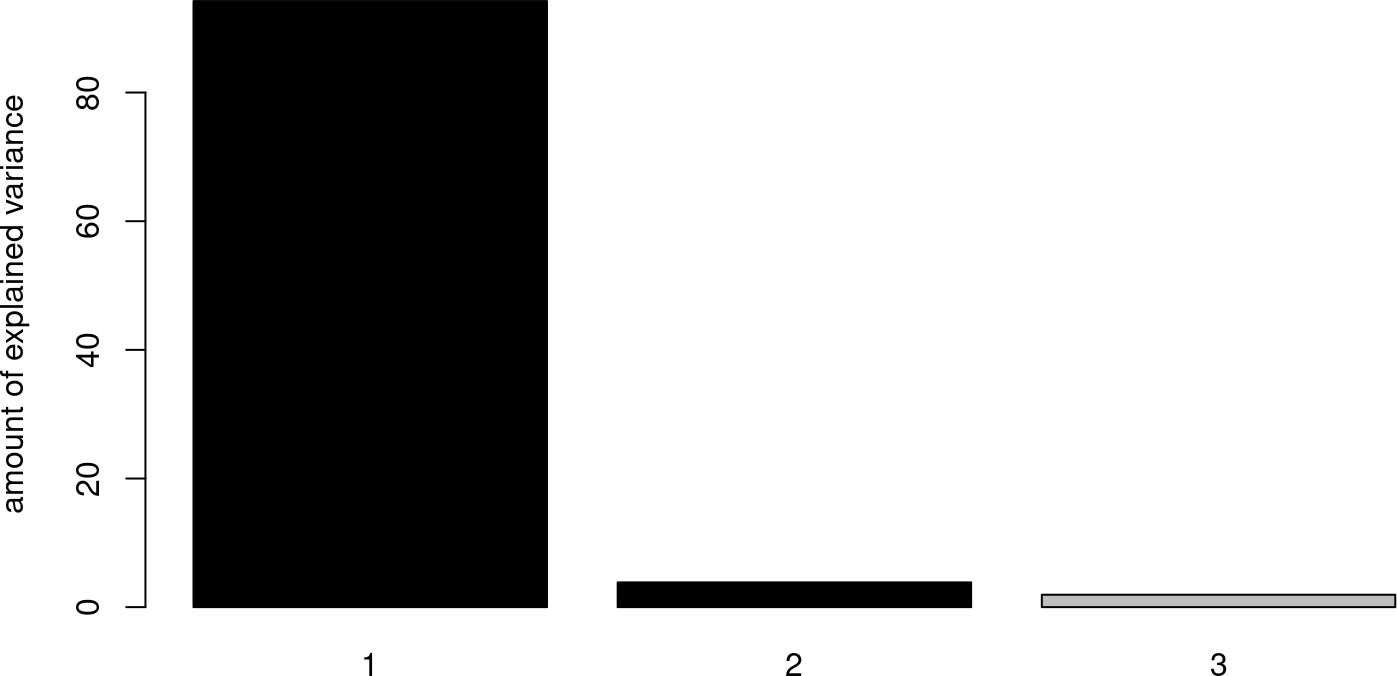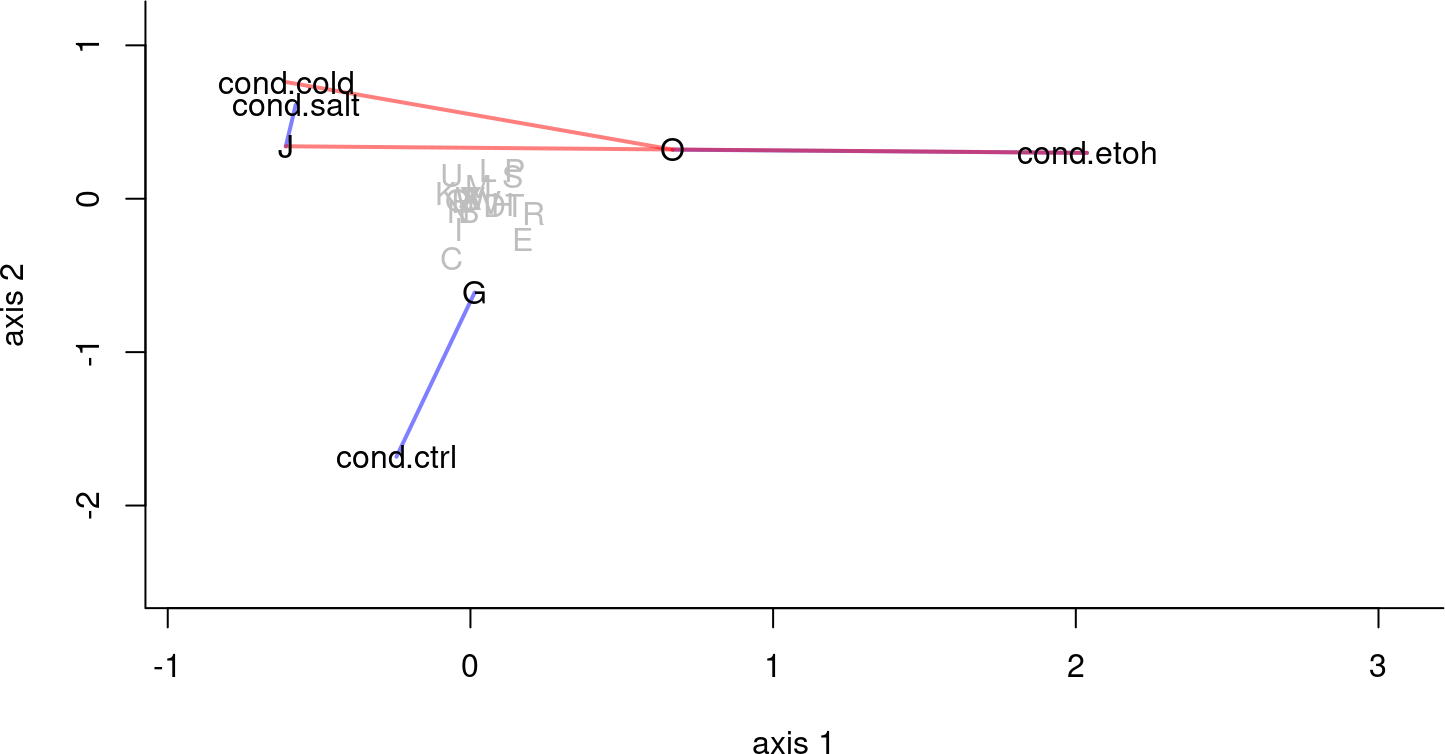# Contents

The R package covRNA provides a convenient and fast interface for testing and visualizing the complex relationships between sample and gene covariates mediated by gene expression data. The fourthcorner analysis tests the statistical significance of sample and gene covariate relationships by permutation tests while the RLQ visualizes relationships within and between sample and gene covariates.

This vignette exemplifies how the covRNA package uses fourthcorner analysis and RLQ for testing and visualizing the relationship between sample and gene covariates mediated by gene expression data. Here, we provide a tutorial with an analysis of an RNA-Seq dataset of Bacillus anthracis that contains various stress conditions as sample covariates and COG annotations (Clusters of Orthologous Groups) as gene covariates. We also show how gene covariates can be assigned to the dataset by using other R packages.

Please install the package as follows.

if (!requireNamespace("BiocManager", quietly=TRUE))
install.packages("BiocManager")
BiocManager::install("covRNA")

# 1 Overview of the Analysis

Gene expression data normally comes with covariates of the samples and of the genes. To analyze associations between sample and gene covariates, the fourthcorner analysis tests the statistical significance of the associations by permutation tests (Legendre et al., 1997) while the RLQ visualizes associations within and between the covariates (Doledec et al., 1996).

The method is based on the powerful fourthcorner and rlq analyses implemented in the R package ade4 package (Dray et al., 2007, and Dray et al., 2014) and used in ecological research for the analysis of species abundance data. We have modified the algorithms of ade4 to make the method suitable to the distributional characteristics of RNA-Seq read counts and microarray intensities and to provide a parallelized high-performance implementation to allow for the analysis of large-scale transcriptomics data. We further supply the user with methods for unsupervised gene filtering and plotting functions to ease the analysis workflow. covRNA can be easily applied to microarray or RNA-Seq data of any organism.

Please refer to the manpages for details about the functions. The package covRNA is implemented to be easily combinable with other packages and objects of the Bioconductor project (Gentleman et al., 2004).

input An ExpressionSet object of the package Biobase can be used as input with assayData containing gene expression data, phenoData the sample covariates and featureData the gene covariates. Alternatively to an ExpressionSet, the three data frames or matrices R, L and Q can be used as input. Here, L contains gene expression data, Q the sample covariates and R the gene covariates.

stat The function stat takes each combination between one sample covariate and one gene covariate and calculates a statistic: If at least one the covariates is quantitative, a correlation coeffcient is calculated. If both covariates are categorical, a Chi-Square test (Fisher, 1922) related statistic is calculated. Significance of the associations is assessed by permutation tests. By default, multiple testing correction according to Benjamini and Hochberg (1995) is applied. The resulting p-values are plotted as cross-tabulation of the sample and gene covariates. By default, red and blue cells show negative and positive significant associations at $$\alpha$$=0.05, respectively.

ord The function ord applies singular matrix ordination to R, L and Q. Correspondence Analysis (CA) is applied to L. Principal Component Analysis (PCA) or Hillsmith Analysis (HA) or Multiple Correspondence Analysis (MCA) are applied to R and Q, depending on the type of variables they contain. Then, the rlq function of the ade4 package is applied and the results can be plotted in various ways.

# 2 Analysis of an RNA-Seq Dataset

Here, an RNA-Seq dataset of Bacillus anthracis (ExpressionSet Baca) containing different stress conditions as sample covariates and COG annotations (Clusters of Orthologous Groups, Tatusov et al., 2000) as gene covariates will be analysed.

## 2.1 Preparation of the dataset

We load the covRNA package and the integrated Baca dataset, which contains the ExpressionSet Baca. The assayData contains deep sequenced RNA-Seq data of 16 samples of B. anthracis (four stress conditions and four replicates per stress condition). The raw sequence reads derive from Passalacqua et al. (2012) and are availaible at Gene Expression Omnibus (GEO, accession number GSE36506). We have mapped, counted and DESeq2 (Love et al., 2014) normalised these counts. The phenoData assigns four stress condition, i.e. ctrl, cold, salt and alcohol stress, to the samples. The featureData assigns COG annotations to the genes.
Alternatively, three matrices or data frames which correspond to assayData, phenoData and featureData, respectively, can be used as input for the following analyses.

library(covRNA)
data(Baca)

## 2.2 Fourthcorner Analysis with stat

We use the function stat for a statistical analysis of the associations between gene and sample covariates.

statBaca <- stat(ExprSet = Baca, npermut = 999, padjust = "BH", nrcor = 2, exprvar = 1)
# or
statBaca <- stat(L = exprs(Baca), R = fData(Baca), Q = pData(Baca), npermut = 999,
padjust = "BH", nrcor = 2, exprvar = 1)

statBaca is an object of type stat. As a list, it saves all results as well as the input of the function. For instance, we can access the adjusted p-values of all covariate combinations and the statistical tests that have been used for their calculations.

ls(statBaca)
adjp <- statBaca$adj.pvalue; adjp tests <- statBaca$stattest; tests

To visualise the results, the stat object can be plotted (Figure 1). The cross-tabulation of the sample and gene functions visualises negative and positive significant associations at $$\alpha$$=0.05. If the plot shall be shown in high quality, we advise to use the default setting pdf=TRUE.

plot(statBaca, xnames = c('cold','ctrl','etoh','salt'), shiftx = -0.1)Figure 1: Cross-tabulation of adjusted p-values of the associations between the sample and gene covariates of the ExpressionSet Baca. Red and blue cells show negative and positive significant associations at $$\alpha$$=0.05, respectively.

## 2.3RLQ with ord

We use the function ord to visualise sample and gene covariates in one ordination plot.

ordBaca <- ord(Baca)

ordBaca is then an object of type ord. Different features of this object can be plotted by using the feature argument of the plot function (see manpages for more information). For instance, we can plot the amount of variance explained by each axis (Figure 2).

plot(ordBaca, feature = "variance")Figure 2: Barplot of the amount of variance explained by each axis of the ordination of Baca. The axes to be taken into account by ordination are shown in black (2 by default).

The first two axes of the RLQ explain a large amount of the variance of the data (93.81$$\%$$ and 4.09$$\%$$, respectively).

## 2.4 Combination of Results

The results of the functions stat and ord can be simultaneously visualised in one ordination plot by the function vis (Figure 3).

vis(Stat = statBaca, Ord = ordBaca, rangex=1.5, rangey=1.5)Figure 3: Simultaneous visualisation of the statistical analysis and of the ordination of Baca. Blue and red lines between the covariates represent positive and negative significant associations, respectively.

In this ordination plot, covariates involved in at least one significant association are shown in black, others are shown in gray. All significant covariates are connected by lines which colour represents positive and negative association, respectively. As expected, positively associated covariates are cluster in the ordination plot, while negatively associated covariates are distantly located at different angles from the origin.

We observe that the first axis seems to be spanned by the difference between the COG classes J and O. The second axis seems to be spanned by the difference between ctrl and the stress conditions. Spatial proximity of cold and salt treatment in the second quadrant suggest that they have similar functional effects on gene expression.

## 2.5 Comparison with Other Methods

To validate our results of the analysis of Baca, we compare them to traditional approaches like hypergeometric test (HG), Mann-Whitney rank test (RANK) und gene set enrichment analysis (GSEA, Subramanian et al., 2005) by using the R package BOG (see Park et al., 2015, for further details).

RANK and GSEA discover class J as significantly enriched (p=6.40e-11 and p=0.02, respectively). HG does not detect any significant gene functions.

# 3 Gene Annotation

If a dataset does not contain gene covariates yet, Bioconductor offers various tools to assign gene covariates to the genes. We propose to use the Bioconductor package biomaRt (Durinck et al., 2009).

> if (!requireNamespace("BiocManager", quietly=TRUE))
+     install.packages("BiocManager")
> BiocManager::install("biomaRt")
> library(biomaRt)

Via the biomaRt package, different databases can be accessed. By using listEnsembl(), for example, all available ENSEMBL databases can be listed (Hubbard et al., 2002). After choosing a database, a dataset can be selected. This dataset will contain different gene functions and other information about genes which can be accessed by listAttributes().

> ensembl <- useEnsembl(biomart = "ensembl")
> listDatasets(ensembl)
> ensemblhuman <- useEnsembl(biomart = "ensembl", dataset = "hsapiens_gene_ensembl")
> listAttributes(ensemblhuman)

If the gene identifiers of the available dataset and the chosen database do not correspond to each other, the Bioconductor package annotate can be used to assign suitable identifiers.

Like this, we get a fully annotated dataset that can be analysed by functions of the covRNA package.

# 4 Installation

The covRNA package is freely available from Bioconductor at https://bioconductor.org/packages/devel/bioc/html/covRNA.html.